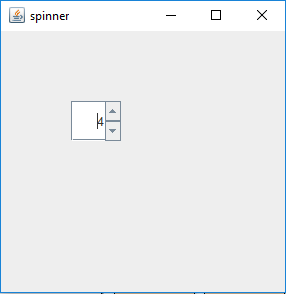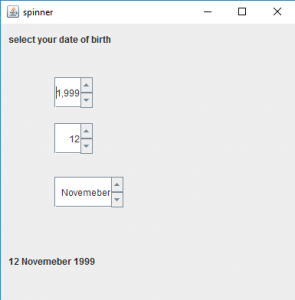# Java Swing | JSpinner

JSpinner is a part of javax.swing package . JSpinner contains a single line of input which might be a number or a object from a ordered sequence . The user can manually type in a legal data into the text field of the spinner . The spinner is sometimes preferred because they do not need a drop down list . Spinners contains a upward and a downward arrow to show the previous and the next element when it is pressed.

Constructors of JSpinner are:

1. JSpinner() : Creates an empty spinner with initial value set to zero and no contraints
2. JSpinner( SpinnerModel model)
creates a spinner with a specified spinner model passed as argument .
3. Commonly used methods are :

1. SpinnerListModel(List l) : creates a spinner model with elements of list l . This spinner model can be used to set as a model for spinner.
2. SpinnerNumberModel(int value, int max, int min, int step) : returns a spinner model whose initial value is set to value, with minimum and maximum value, and a definite step value.
3. setValue(Object v) : sets the value of the spinner to the object passed as argument.
4. getValue() : returns the current value of the spinner.
5. getPreviousValue() : returns the previous value of the spinner.
6. getNextValue() : returns the next value of the spinner.

1. Program to create a simple JSpinner

 `// java Program to create a ` `// simple JSpinner ` `import` `java.awt.event.*; ` `import` `javax.swing.*; ` `import` `java.awt.*; ` `class` `spinner ``extends` `JFrame { ` `    ``// frame ` `    ``static` `JFrame f; ` ` `  `    ``// default constructor ` `    ``spinner() ` `    ``{ ` `    ``} ` ` `  `    ``// main class ` `    ``public` `static` `void` `main(String[] args) ` `    ``{ ` `        ``// create  a new frame ` `        ``f = ``new` `JFrame(``"spinner"``); ` ` `  `        ``// create a JSpinner ` `        ``JSpinner s = ``new` `JSpinner(); ` ` `  `        ``// set Bounds for spinner ` `        ``s.setBounds(``70``, ``70``, ``50``, ``40``); ` ` `  `        ``// set layout for frame ` `        ``f.setLayout(``null``); ` ` `  `        ``// add panel to frame ` `        ``f.add(s); ` ` `  `        ``// set frame size ` `        ``f.setSize(``300``, ``300``); ` ` `  `        ``f.show(); ` `    ``} ` `} `

Output :2. Program to create a JSpinner and add ChangeListener to it ; Program to select your date of birth using JSpinner)

 `// Java program to select your ` `// date of birth using JSpinner ` `import` `java.awt.event.*; ` `import` `javax.swing.*; ` `import` `java.awt.*; ` `import` `javax.swing.event.*; ` `class` `spinner1 ``extends` `JFrame ``implements` `ChangeListener { ` `    ``// frame ` `    ``static` `JFrame f; ` ` `  `    ``// label ` `    ``static` `JLabel l, l1; ` ` `  `    ``// spinner ` `    ``static` `JSpinner s, s1, s2; ` ` `  `    ``// default constructor ` `    ``spinner1() ` `    ``{ ` `    ``} ` ` `  `    ``// main class ` `    ``public` `static` `void` `main(String[] args) ` `    ``{ ` `        ``// create an object of the class ` `        ``spinner1 sp1 = ``new` `spinner1(); ` ` `  `        ``// create  a new frame ` `        ``f = ``new` `JFrame(``"spinner"``); ` ` `  `        ``// create a label ` `        ``l = ``new` `JLabel(``"select your date of birth"``); ` `        ``l1 = ``new` `JLabel(``"1 January 2000"``); ` ` `  `        ``// create a JSpinner with a minimum, maximum and step value ` `        ``s = ``new` `JSpinner(); ` `        ``s1 = ``new` `JSpinner(``new` `SpinnerNumberModel(``1``, ``1``, ``31``, ``1``)); ` ` `  `        ``// setvalue of year ` `        ``s.setValue(``2000``); ` ` `  `        ``// store the months ` `        ``String months[] = { ``"January"``, ``"February"``, ``"March"``, ` `        ``"April"``, ``"May"``, ``"June"``, ``"July"``, ``"August"``,  ` `        ``"September"``, ``"October"``, ``"Novemeber"``, ``"December"` `}; ` ` `  `        ``// create a JSpinner with list values ` `        ``s2 = ``new` `JSpinner(``new` `SpinnerListModel(months)); ` ` `  `        ``// add change listener to spinner ` `        ``s.addChangeListener(sp1); ` `        ``s1.addChangeListener(sp1); ` `        ``s2.addChangeListener(sp1); ` ` `  `        ``// set Bounds for spinner ` `        ``s.setBounds(``70``, ``70``, ``50``, ``40``); ` `        ``s1.setBounds(``70``, ``130``, ``50``, ``40``); ` `        ``s2.setBounds(``70``, ``200``, ``90``, ``40``); ` ` `  `        ``// setbounds for label ` `        ``l.setBounds(``10``, ``10``, ``150``, ``20``); ` `        ``l1.setBounds(``10``, ``300``, ``150``, ``20``); ` ` `  `        ``// set layout for frame ` `        ``f.setLayout(``null``); ` ` `  `        ``// add label ` `        ``f.add(l); ` `        ``f.add(l1); ` `        ``f.add(s); ` `        ``f.add(s1); ` `        ``f.add(s2); ` ` `  `        ``// add panel to frame ` `        ``f.add(s); ` ` `  `        ``// set frame size ` `        ``f.setSize(``400``, ``400``); ` ` `  `        ``f.show(); ` `    ``} ` ` `  `    ``// if the state is changed ` `    ``public` `void` `stateChanged(ChangeEvent e) ` `    ``{ ` `        ``l1.setText(s1.getValue() + ``" "` `+ s2.getValue() + ``" "` `+ s.getValue()); ` `    ``} ` `} `

Output :Note : This program will not run in an online compiler please use an Offline IDE

Attention reader! Don’t stop learning now. Get hold of all the important Java and Collections concepts with the Fundamentals of Java and Java Collections Course at a student-friendly price and become industry ready.

My Personal Notes arrow_drop_upCheck out this Author's contributed articles.

If you like GeeksforGeeks and would like to contribute, you can also write an article using contribute.geeksforgeeks.org or mail your article to contribute@geeksforgeeks.org. See your article appearing on the GeeksforGeeks main page and help other Geeks.

Please Improve this article if you find anything incorrect by clicking on the "Improve Article" button below.